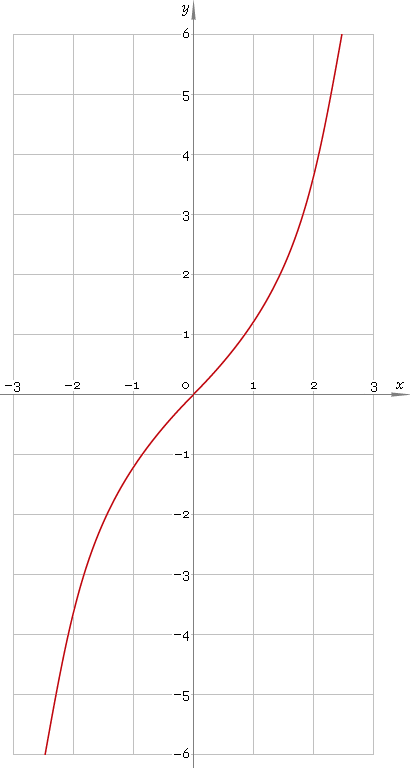The Art of Mathematics

# sinh or sh — hyperbolic sine function

## 1. Definition

Hyperbolic sine is defined as

sinhx ≡ (ex − ex) /2

## 2. Graph

Hyperbolic sine is antisymmetric function defined everywhere on real axis. Its graph is depicted below — fig. 1.Fig. 1. Graph of the hyperbolic sine function y = sinhx.

Function codomain is entire real axis.

## 3. Identities

Base:

cosh2x − sinh2x = 1

Relation to exponential function:

sinhx + coshx = ex
coshx − sinhx = ex

By definition:

sinhx ≡ 1 /cschx

Property of antisymmetry:

sinh−x = −sinhx

Half-argument:

sinh(x/2) = √[(coshx − 1) /2]
sinhx = 2 tanh(x/2) /[1 − tanh2(x/2)]

Double argument:

sinh(2x) = 2 sinhx coshx

Triple argument:

sinh(3x) = 4 sinh3x + 3 sinhx

sinh(4x) = 4 sinh3x coshx + 4 sinhx cosh3x

Power reduction:

sinh2x = (cosh(2x) − 1) /2
sinh3x = (sinh(3x) − 3 sinhx) /4
sinh4x = (cosh(4x) − 4 cosh(2x) + 3) /8
sinh5x = (sinh(5x) − 5 sinh(3x) + 10 sinhx) /16

Sum and difference of arguments:

sinh(x + y) = sinhx coshy + coshx sinhy
sinh(xy) = sinhx coshy − coshx sinhy

Product-to-sum:

sinhx sinhy = [cosh(x + y) − cosh(xy)] /2
sinhx coshy = [sinh(x + y) + sinh(xy)] /2

Sum-to-product:

sinhx + sinhy = 2 sinh[(x + y) /2] cosh[(xy) /2]
sinhx − sinhy = 2 sinh[(xy) /2] cosh[(x + y) /2]

## 4. Derivative and indefinite integral

Hyperbolic sine derivative:

sinh′x = coshx

Indefinite integral of the hyperbolic sine:

∫ sinhx dx = coshx + C

where C is an arbitrary constant.

## 5. How to use

To calculate hyperbolic sine of the number:

``sinh(−1);``

To get hyperbolic sine of the complex number:

``sinh(−1+i);``

To get hyperbolic sine of the current result:

``sinh(rslt);``

To get hyperbolic sine of the number z in calculator memory:

``sinh(mem[z]);``

## 6. Support

Hyperbolic sine of the real argument is supported in free version of the Librow calculator.

Hyperbolic sine of the complex argument is supported in professional version of the Librow calculator.